# OPTIMIZATION BASED CONTROL STRATEGIES OF HYBRID ELECTRIC VEHICLES

СТРАТЕГИИ УПРАВЛЕНИЯ ГИБРИДНЫМИ ЭЛЕКТРОМОБИЛЯМИ НА ОСНОВЕ ОПТИМИЗАЦИИ
Цитировать:
Usmanov U., Karimov A. OPTIMIZATION BASED CONTROL STRATEGIES OF HYBRID ELECTRIC VEHICLES // Universum: технические науки : электрон. научн. журн. 2023. 1(106). URL: https://7universum.com/ru/tech/archive/item/14821 (дата обращения: 03.06.2023).
Прочитать статью:

ABSTRACT

The increasing number of environmental concerns, raising as a result of greenhouse gas emissions in transportation sector made it almost essential to switch to alternative fuels or electrifying/hybridizing the propulsion system. Hybrid electric vehicle (HEV) is one possible solution to meet the requirements and limits of exhaust pollutant and greenhouse gas emissions. Control strategies or energy management strategy (EMS) ensures the optimal distribution of required power between different power sources of of hybrid vehicles. The proper design of EMS of hybrid vehicles ensures the minimization of both fuel consumption and engine emissions. In this article, various control strategies (EMS) based on optimization are discussed. Both on-line and global optimization strategies of HEV, their potential as well as advantages and disadvantages are provided and thoroughly described.

АННОТАЦИЯ

Растущее количество экологических проблем, возникающих в результате выбросов парниковых газов в транспортном секторе, сделало практически необходимым переход на альтернативные виды топлива или электрификацию/гибридизацию силовой установки. Гибридный электромобиль (ГЭМ) — это одно из возможных решений, позволяющее удовлетворить требования и ограничить выбросы загрязняющих выхлопных газов и парниковых газов. Стратегии управления или стратегии управления энергопотреблением (СУЭП) обеспечивают оптимальное распределение требуемой мощности между различными источниками питания гибридных транспортных средств. Надлежащая конструкция СУЭП гибридных автомобилей обеспечивает минимизацию как расхода топлива, так и выбросов двигателя. В данной статье обсуждаются различные стратегии управления (СУЭП), основанные на оптимизации. Представлены и подробно описаны как онлайновые, так и глобальные стратегии оптимизации ГАМ, их потенциал, а также преимущества и недостатки.

Keywords: Hybrid electric vehicles (HEV), optimization-based energy management strategies (EMS), fuel consumption minimization.

Ключевые слова: Гибридные автомобили (ГАМ), стратегии управления энергопотреблением (СУЭП), основанный на оптимизации, минимизация расхода топлива.

1. Introduction

In order to ensure that the energy provided to the drivetrain is adequate in accordance with the demand or load power, HEV is studied in terms of the many energy sources that must be correctly controlled. This is because, depending on the situation, electricity could be produced using each energy source, or a combination of them. At other times, both the battery can be charged, while internal combustion engine (ICE) can provide power both for charging and propulsion and at other times, the energy is provided by both the battery and ICE . The broad guidelines for EMS development are founded on a number of fundamental rules, including the optimization of both ICE and battery systems. These rules force all energy sources to operate at optimum operating line (OOL), i.e., maximum efficiency working point; minimize the dynamics of ICE as well as optimization of ON/OFF periods; battery operates in charge-sustaining mode to prolong the battery life and to allow electric motor (EM) to work efficiency; intensification of regenerative braking based on driver’s action . Overall, we can classify 5 operating modes of hybrid vehicles: charging while driving, energy regeneration during braking, battery charging, power starving and batter drive (Figure 1).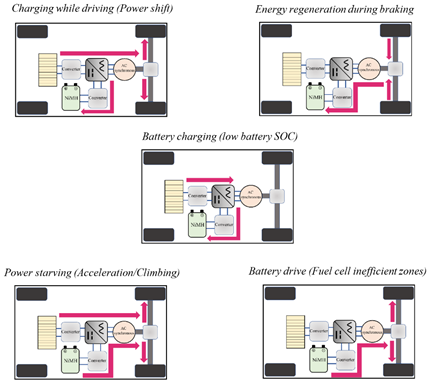Figure 1. Operating modes of hybrid vehicles

EMSs are divided into two major categories: rule-based strategies and optimization-based strategies. Rule-based strategies can be further divided into deterministic and fuzzy-logic rule-based strategies, while optimization-based strategies are divided into instantaneous (real-time) optimization, global optimization and model predictive control (Figure 2). Additionally, all EMSs are categorized into two groups (offline and online) based on the usage of real-time implementation because the goal of building any EMS is to utilize it in practical applications or to serve as a benchmark to assess the efficacy of other techniques .

Optimization based control strategies are based on the minimization of cost function by means of analytical or numerical operations . The cost function takes into account several parameters. These might be fuel consumption, efficiency and emission data. The solution of the problem is optimum either globally or locally (only for a given set of input variables). When the driving pattern is known a priori, which is not achievable in practical application, the global optimum solution can be determined. As a result, it cannot be used in actual controllers, although online optimization can be carried out via instantaneous cost function minimization . Using instantaneous (real-time) optimization procedures, the best solution is reached by minimizing the instantaneous cost function and using the most recent input data. The data for the whole driving conditions, pattern, and time history of other factors is needed for global optimization strategies.

2. Equivalent consumption minimization strategy

This technique was first proposed by Paganelli et al.  and aims at minimization of cost function, which is equivalent fuel consumption. The idea is that electrical energy can be equivalently converted to fuel consumption. The total fuel consumption, which is the cost function is the sum of direct consumption, which comes from ICE and equivalent battery fuel consumption. The calculation of equivalent fuel consumption of batteries is performed by making an assumption that SOC variation in the future is compensated by ICE.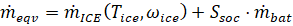(1)

Where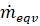is equivalent (total) fuel consumption rate;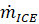-ICE fuel consumption rate, which depends on engine torque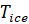and speed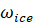;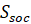-penalty function due to battery SOC stabilization;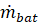-equivalent battery fuel consumption.

Average efficiencies of power units, fuel consumption rate and mean power are required to calculate the equivalent fuel consumption of batteries . The equivalent fuel consumption is calculated using ECMS based on the online measurements of the quantities on board and the current system condition. In order to obtain an optimal solution, no prior knowledge of driving behavior is necessary, and can be applied online. It reduces a global optimization problem into an instantaneous minimization problem and provides the optimum solution at each time instant .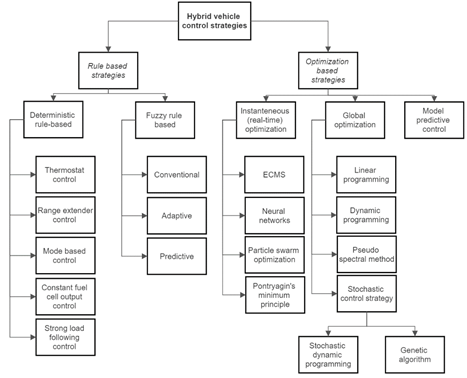Figure 2. Classification of control strategies of HEV

3. Neural networks

A computer technology known as an artificial neural network (ANN) can replicate a biological brain and is intended to carry out a given task without actually knowing the physical model of a system. ANN creates output data by learning from the previous experience. Neural networks are trained by constructing probability-weighted associations between the two that are then stored within the net's data structure while processing samples that each have a known "input" and "output" . One must choose from a list of approved models and related methodologies for ANN training. The network's processed output and the desired output are then frequently compared using an example as a starting point. The network then adjusts its weighted associations utilizing a learning technique and this error value. The neural network will produce output that is increasingly comparable to the goal output as modifications are made over time . These modifications can be made a sufficient number of times before the training can be stopped under certain conditions. There are three or more interconnected layers in an ANN. Neurons in the input layer make up the first layer. These neurons transmit information to hidden layers, which then transmit the final output information to the final output layer .

4. Particle swarm optimization

A computer heuristic technique known as particle swarm optimization (PSO) iteratively improves the predicted solution to optimize the objective function. The movement of particles (also known as the population or candidate solution) inside the search space is controlled by mathematical relationships over their position and velocity . In PSO, particles use both their own best-known positions and the positions of the entire swarm to move through a search space. The improved placements, once determined, will control the motion of the swarm particles. These approaches should cause the swarm to navigate toward the best solution. Repeating the procedure, however, does not guarantee a positive result. PSO is a metaheuristic approach since it has a broad search space for potential solutions and makes few to no assumptions about the problem it is trying to solve. PSO does not, however, guarantee the best result . More particular, PSO does not use the gradient of the improved problem, which means that, in contrast to conventional optimization approaches, PSO does not need that the improved problem be differentiable .

5. Pontryagin’s minimum principle

It is a generalization of Euler-Lagrange equations, that takes into account constraints on the control input. It provides a control for a dynamical system to be takes from one state to another when the constraints for a state or input control are present. It claims that in order to solve the two-point boundary value problem known as the "Hamiltonian system," which is a two-point optimum control problem, both the optimal state trajectory and any optimal control are required .

Control Hamiltonian function H can be constructed by appending the state equation to the integrand L using the Lagrange multipliers, λ(t) follows :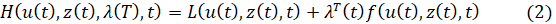Where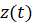is the optimal control, and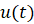is the corresponding optimal state. Then, there exists a continuous function λ, called adjoint function, which is the solution of the adjoint equation.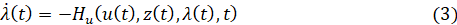Where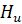is differential of the Hamiltonian function. The adjoint function is a Lagrange multiplier that informs the optimization problem of the state equation constraint. Hamiltonian function is minimized by z(t), corresponding optimal state u(t) and adjoint λ(t) according to the Pontryagin’s minimum principle.

6. Linear programming

When the requirements are expressed as linear relationships in a mathematical model, linear programming (LP) is a special case of mathematical optimization that looks for the optimal solution. Relationships based on linear equality and inequality express the limitations, and linear relationships are used to characterize the objective function. Linear programming problems are a major class of optimization problems because they require the location of the feasible region and optimization of the solution to have the highest or lowest value of the function . Different approaches, such as the graphical method, the simplex method, or the use of additional tools, can be used to tackle LP problems. Some assumptions must be taken into account while using this technique: expression of constraints in quantitative terms; presence of linear relationships between the constraints and objective function; optimization of linear (objective) function . The components of this technique are objective function, constraints, decision variables, data. The formulation of LP method is(4)

The constraints are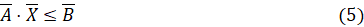In reality, improving fuel economy is a convex nonlinear optimization problem that is ultimately approximated by linear programming. Linear programming is mainly used in series HEVs to increase fuel efficiency. A global optimal solution is the outcome of the LP optimization method.

7. Dynamic programming

Dynamic programming (DP) is an optimization technique that transforms a complex problem into a series of simpler ones. A broad framework for studying a wide variety of problem types is provided by dynamic programming. This framework allows for the use of a range of optimization strategies to address specific issues with a more general formulation . It has the benefit of being applicable to problems with constraints and without constraints as well as linear and nonlinear systems. It should be emphasized that the overall problem depends on the optimal solution to its subproblems. The appropriate division of optimization problems into several levels, each of which is solved one level at a time is a crucial component of DP .

However, the main disadvantage of this technique is the "curse of dimensionality", which increases the computational difficulty and restricts the use of the method to complex systems. The DP algorithm cannot be used in real time because it requires prior knowledge of the driving cycle. Its outputs, however, can be utilized to design and fine-tune real controllers.

8. Pseudo-spectral method

Combining theoretical and computational approaches, pseudo-spectral optimization technology addresses optimization issues. Pseudospectral optimal control is a term that covers a variety of ideas . Among these are the Ross-Fahroo pseudospectral method, the Gauss pseudospectral method, the Legendre pseudospectral method, the flat pseudospectral method, the Bellman pseudospectral approach, and many others . To address an optimal control problem, it is necessary to approximate the integration in the cost function, the differential equation of the control system, and the state-control constraints . The best approximation technique should be effective for all three approximate objectives. It's possible that a method, such as an efficient ODE solver, that works well for one of them may not work well for the other two. The best option for satisfying these requirements is to employ PS methods because they are efficient at approximating all three mathematical objects . A group of properly selected quadrature nodes are utilized in a pseudospectral approach to approximate the continuous functions. The quadrature nodes are determined by the related orthogonal polynomial basis used for the approximation. In PS optimal control, Legendre and Chebyshev polynomials are widely used.

9. Stochastic control strategy

The stochastic approach provides a framework for modelling optimization problems that include uncertainty. This approach is used to formulate an infinite-horizon stochastic dynamic optimization problem. A stochastic Markov process is used to model the driver's power request. The Markov driver model generates the probability distribution for the anticipated future power requests and predicts what they will be. This prediction does not depend on the decisions made in the past . The best control strategy is then chosen using stochastic dynamic programming. The derived control law, which takes the form of a stationary full-state feedback, is directly implementable. It is found that the derived SDP control strategy outperforms a suboptimal rule-based control approach learned from deterministic DP findings. The stochastic technique optimizes the control strategy over a family of diverse driving cycles as opposed to deterministic optimization over a single DC . Stochastic control strategy is further divided into stochastic dynamic programming and genetic algorithms.

Random variables are used to represent the optimization problem in stochastic dynamic programming (SDP). State or decision functions are formulated in terms of probability functions in SDP. This demands a lot of computational work.

Heuristic search algorithms are used to optimise the search issues in the case of genetic algorithms (GA). The underlying idea of GA is derived from Darvin's theory of evolution. As a beginning point, a preliminary set of answers from a certain population should be offered. The replies from one population are used depending on their capacity to produce new populations. Since the best solutions have a higher likelihood of developing than the worst ones, the process is kept going until the desired state is achieved.

10. Model predictive control

Model predictive control (MPC) uses a model to predict the future outputs based on previous and current values. The models, that are used in MPC describe the behaviour of complex and simple dynamic systems. MPC models forecast the change in the modeled system’s dependent variables that will result from changes in the independent variables . Future changes in the dependent variables are calculated using MPC using the current plant data, the present dynamic state of the process, the MPC models, and the process variable targets and limits. These adjustments are calculated to respect limitations on both the independent and dependent variables and keep the dependent variables around their desired values. When a subsequent modification is required, the MPC normally repeats the calculation after sending out the first change for each independent variable to be applied . Several strategies can be utilized when linear models are insufficiently accurate to capture the true process nonlinearities. To lessen nonlinearity, the process variables may occasionally be changed prior to and/or following the linear MPC model. Nonlinear MPC, which employs a nonlinear model directly in the control application, may control the process. The main advantage of MPC is that it enables timeslot optimization while taking future timeslots into consideration. This is accomplished by repeatedly optimizing a finite time horizon while only using the current time slot for implementation.

11. Conclusion

According to earlier research, EMS is a crucial component of a hybrid vehicle. The EMS is the controller that decides which energy source will supply the required amount of power for the particular mode of operation. Since most EMS types ignore battery deterioration, EMS optimization for a HEV is essential and required. This article has analyzed and addressed earlier investigations into the improvement of HEV EMS. Despite the fact that numerous research on EMS optimization have been presented, the most of them are restricted to simulation and do not include real-time applications or experimental setup validation. As a result, an experimental setup or real-time application must be used to validate the optimization of the EMS simulation.

References:

1. Aishwarya Panday and Hari Bansal. «A Review of Optimal Energy Management Strategies for Hybrid Electric Vehicle». In: (Dec. 2014)
2. D.E Kirk. Optimal control theory: an introduction. [Courier Corporation]. 2012
3. Fariba Fahroo and I Ross. «Advances in Pseudospectral Methods for Optimal Control». In: AIAA Guidance, Navigation and Control Conference and Exhibit (Aug. 2008).
4. G. Paganelli, Sebastien Delprat, Thierry-Marie Guerra, J. Rimaux, and JeanJacques Santin. «Equivalent consumption minimization strategy for parallel hybrid powertrains». In: vol. 4. Feb. 2002, 2076–2081 vol.4.
5. I. Ross and R. Proulx. «Further Results on Fast Birkhoff Pseudospectral Optimal Control Programming». In: Journal of Guidance, Control, and Dynamics 42 (Apr. 2019), pp. 1–7.
6. I. Ross and Mark Karpenko. «A review of pseudospectral optimal control: From theory to flight». In: Annual Reviews in Control 36 (Dec. 2012), pp. 182– 197.
7. Liu W. Introduction to Hybrid Vehicle System Modeling and Control. John Wiley Sons, Inc, 2013
8. M. Sabri, Kumeresan A. Danapalasingam, and Mohd Rahmat. «A review on hybrid electric vehicles architecture and energy management strategies». In: Renewable and Sustainable Energy Reviews 53 (Jan. 2016), pp. 1433–1442.
9. Siang Tie and Chee Wei Tan. «A review of energy sources and energy management ystem in electric vehicles». In: Renewable and Sustainable Energy Reviews 20 (Apr. 2013), pp. 82–102.
10. Sara Luciani and Andrea Tonoli. «Control Strategy Assessment for Improving PEM Fuel Cell System Efficiency in Fuel Cell Hybrid Vehicles». In: Energies 15 (Mar. 2022), p. 2004.
11. Sukanta Nayak. «Chapter seven - Dynamic programming». In: Fundamentals of Optimization Techniques with Algorithms. Ed. by Sukanta Nayak. Academic Press, 2020, pp. 191–221
12. Umidjon Usmanov. Control strategy optimization of Toyota Mirai based fuel cell hybrid electric vehicles (Master’s thesis). Politecnico di Torino, October 2022.
13. У.Р.Усманов. Обзор стратегий управления энергопотреблением гибридных автомобилей, основанные на логических правилах. Universum: Технические науки 10-2 (103), 2022, стр 57-61.
14. What is a neural network. https://www.tibco.com/reference-center/what-is-a-neural-network
15. Xiao-Hong Yuan, Guo-Dong Yan, Hong-Tao Li, Xun Liu, Chu-Qi Su, and YiPing Wang. «Research on energy management strategy of fuel cell–battery–supercapacitor passenger vehicle». In: Energy Reports 8 (2022). 2021 The 8th International Conference on Power and Energy Systems Engineering, pp. 1339–1349.
16. Yanjun Huang, Hong Wang, Amir Khajepour Amir, Bin Li, Jie Ji, Kegang Zhao, and Chuan Hu. «A review of power management strategies and component sizing methods for hybrid vehicles». In: Renewable and Sustainable Energy Reviews 96 (Nov. 2018), pp. 132–144.
Информация об авторахMaster of technical sciences, lecturer in Mechanical and Aerospace Engineering department in Turin Polytechnic University in Tashkent, Republic of Uzbekistan, Tashkent

магистр технических наук, преподаватель кафедры “Технология машиностроения и авиакосмический инжиниринг” в Туринском политехническом университете в г. Ташкенте, Республика Узбекистан, г. ТашкентMaster’s students in 70711401-Technological processes and automation of production at “Tashkent Institute of Irrigation and Agricultural Mechanization Engineers” National Research University, Republic of Uzbekistan, Tashkent

магистрант по специальности 70711401- Автоматизация производства и технологических процессов национального исследовательского университета «Ташкентский институт инженеров ирригации и механизации сельского хозяйства», Республика Узбекистан, г. Ташкент

Журнал зарегистрирован Федеральной службой по надзору в сфере связи, информационных технологий и массовых коммуникаций (Роскомнадзор), регистрационный номер ЭЛ №ФС77-54434 от 17.06.2013
Учредитель журнала - ООО «МЦНО»
Главный редактор - Ахметов Сайранбек Махсутович.
Top